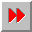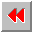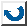EMA-XPS Online

FORMAT

```FORMAT
======

syntax: (format <stream> <format-string> {<arg> ...})

This function is used to produce a formatted output.
The format directives consist of a tilde (~),
optional prefix parameters separated by commas,
optional colon (:) and at-sign (@) modifiers,
and a single character indicating what kind of
directive this is.

~A     Ascii. An <arg>, any Lisp object, is printed
without escape characters. The output
is not suitable for input to read.
~S     Symbolic-expression. This is just like ~A,
but <arg> is printed with escape
characters. The output is therefore
~D     Decimal. An <arg>, which should be an integer,
~B     Binary. This is just like ~D but prints in
~O     Octal. This is just like ~D but prints in
~X     Hexadecimal. This is just like ~D but prints
decimal.
~F     Fixed-format floating-point. The next <arg>
is printed as a floating point number.
The full form is ~w,d,kF. The
parameter w is the width of the field
to be printed. d is the number of
digits to print after the decimal
point. k is a scale factor that
defaults to zero.
~E     Exponential floating-point. The next <arg> is
printed as a floating point number in
exponential notation. The full form is
~w,d,e,kE. e is the number of digits
to use when printing the exponent.
~\$     Dollars floating-point. The next argument is
printed as a floating-point number in
fixed-format notation. This format is
particularly convenient for printing
a value as dollars and cents. The full
form is ~d,n,w\$. n is the minimum
number of digits to print before the
decimal point (default value 1)
~%     This outputs a #\newline character, thereby
terminating the current output line
and beginning a new one.
~|     This outputs a page separator character, if
possible.
~T     Tabulate. This spaces over to a given column.
~colnum,colincT will output sufficient
spaces to move the cursor to column
'colnum'.
~~     This outputs a tilde.
~<nl>  Tilde immediately followed by a newline
ignores the newline and any following
non-newline whitespace characters.

```EMA-XPS Online